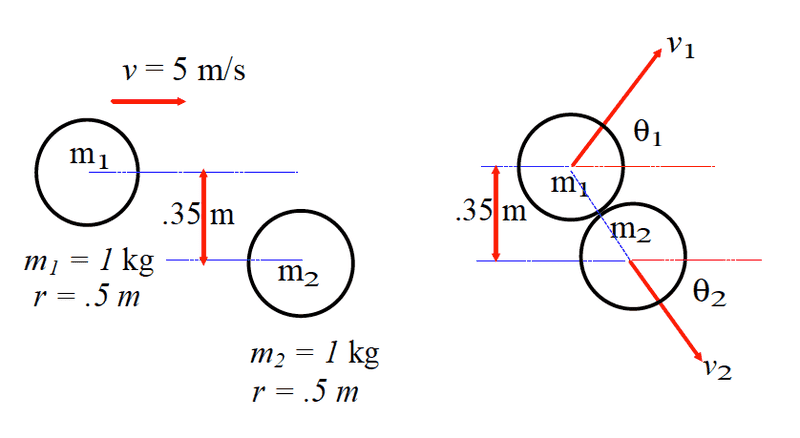# Elastic Collision with objects of equal masses

## Homework Statement

Solve for the Velocity of each mass after the collision.

Pi = Pf
Ki = Kf

## The Attempt at a Solution

Okay so I've tried setting up a system of equations to solve for the unknown velocity and angles. The problem is that I can't seem to find either unknown angle. I know that momentum for each axis must be conserved and that total energy must be conserved. That gives me three equations but I can't figure out how to solve for both the velocity and the angles. I do know that θ1 + θ2 = 90°## Answers and Replies

Orodruin
Staff Emeritus
Science Advisor
Homework Helper
Gold Member
In which direction is the force acting on ##m_2## during the collision directed?

45° below the horizon? I know it has something to do with this I'm just at a complete loss.

Orodruin
Staff Emeritus
Science Advisor
Homework Helper
Gold Member
45° below the horizon? I know it has something to do with this I'm just at a complete loss.

What makes you say 45°? What is your rationale behind this? Can you make a geometric argument?

I can't but I think I see what your getting at. Because we know the distance between the center of the circles we should be able to calculate the angle. Using (.35/2)m and radius of .5m I get an angle 20.5 degrees below the horizon.

Orodruin
Staff Emeritus
Science Advisor
Homework Helper
Gold Member
So, this gives you an angle. What will you do with it?

It gives me the angle of ∅2 and by extension ∅1. Because there are no other forces acting on m2 the impulse is in the same direction as the force, which also means that the momentum will be in the same direction. From there, using the conservation of momentum in both the x and y direction I can easily solve for the magnitude of each velocity.

Orodruin
Staff Emeritus
Science Advisor
Homework Helper
Gold Member
Indeed, too bad I am always unable to put this into practise at the pool table ...(Although billiard ball collisions obviously are not elastic ...)